Post

# How to generate documentations according to your project rapidly

• 7.6k
• 0
• 0

It's possible to generate documents according to your project as clean and fast as possible. In fact, Visual studio offers the possibility to insert tags into the code editor as mentioned below: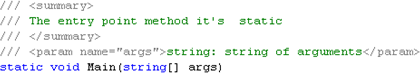Figure 1

As you can see every class member should be decorated by those tags in order to be documented later. Documentation could be ventilated toward HTML files using the XSLT transformation in order to obtain a sort of a web site that could be used locally to browse through the details of your project. Indeed, this sort of documentation is called NDOC it's very similar to the JAVA DOC if you're coming from Java developments back ground.

Walkthrough:

I propose this walkthrough to illustrate how to build such documentation:

First, create a new console application and add this code:

using System;

using System.Collections.Generic;

using System.Text;

/** The namespace DocumentGenerate   */

namespace DocumentGenerate

{

/// <summary>

/// This is the Program class that contains the main method

/// </summary>

class Program

{

/// <summary>

/// The entry point method it's  static

/// </summary>

/// <param name="args">string: string of arguments</param>

static void Main(string[] args)

{

Arithmetic Ar = new Arithmetic(36, 3);

int sum = Ar.Sum();

int dif = Ar.Diff(true);

int mul = Ar.Mult();

double div = Ar.Div(true);

Console.WriteLine(string.Format("Numners are {0} and {1}",Ar.a,Ar.b));

Console.WriteLine(string.Format("{0} + {1} = {2}",Ar.a,Ar.b,Ar.Sum()));

Console.WriteLine(string.Format("{0} - {1} = {2}", Ar.a, Ar.b, Ar.Diff(true)));

Console.WriteLine(string.Format("{0} * {1} = {2}", Ar.a, Ar.b, Ar.Mult()));

Console.WriteLine(string.Format("{0} / {1} = {2}", Ar.a, Ar.b, Ar.Div(true)));

}

}

/// <summary>

/// Arithmetic: This class helps to perform basic arithmetic operations

/// </summary>

public class Arithmetic

{

/// <summary>

/// Integer : Private field that represents the first operand

/// </summary>

private int _a;

/// <summary>

/// Integer : Private field that represent the second operand

/// </summary>

private int _b;

/// <summary>

/// Constructor: Default constructor

/// </summary>

public Arithmetic() { }

/// <summary>

/// Constructor: Constructor that recieves two operands

/// </summary>

/// <param name="a">a : First operand</param>

/// <param name="b">b : Second operand</param>

public Arithmetic(int a, int b)

{

this.a = a;

this.b = b;

}

/// <summary>

/// Integer: This is the first operand public propertie

/// </summary>

public int a

{

get { return _a; }

set {_a = value; }

}

/// <summary>

/// Integer: This is the second operand public propertie

/// </summary>

public int b

{

get { return _b; }

set { _b = value; }

}

/// <summary>

/// </summary>

/// <returns>Integer: The sum of to integer</returns>

public int Sum()

{

return a + b;

}

/// <summary>

/// Method:

/// </summary>

/** <param name="FirstMinusSecond">Boolean : Indicates if

* the a should be at first position</param>*/

/// <returns>Integer: Difference between to integers</returns>

public int  Diff(bool FirstMinusSecond)

{

FirstMinusSecond = true;

if (FirstMinusSecond)

return a - b;

else

return b - a;

}

/// <summary>

/// Method : Multiply two integers

/// </summary>

/// <returns>Integer: Multiplication of two integers</returns>

public int Mult()

{

return a * b;

}

/// <summary>

/// Method : Divide one integer by another integer

/// </summary>

/** <param name="FirstDivSecond">Boolean: Indicates if

* the first operand should be a

* </param>*/

/// <returns>Double: Division of two integers</returns>

public double Div(bool FirstDivSecond)

{

FirstDivSecond = true;

if (FirstDivSecond)

{

try

{

return a / b;

}

catch(DivideByZeroException caught)

{

Console.WriteLine(caught.Message);

return 0;

}

}

if (!FirstDivSecond)

{

try

{

return b / a;

}

catch (DivideByZeroException caught)

{

Console.WriteLine(caught.Message);

return 0;

}

}

else return 0;

}

}
}

This project is composed by two classes, the class Program and the class Arithmetic that can perform some arithmetic operations. In order to generate an xml that contains the project documentation do as follow:

1. Go to solution explorer then right click the project and select properties: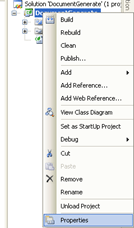Figure 2

2. A window appears as below, select the build tag: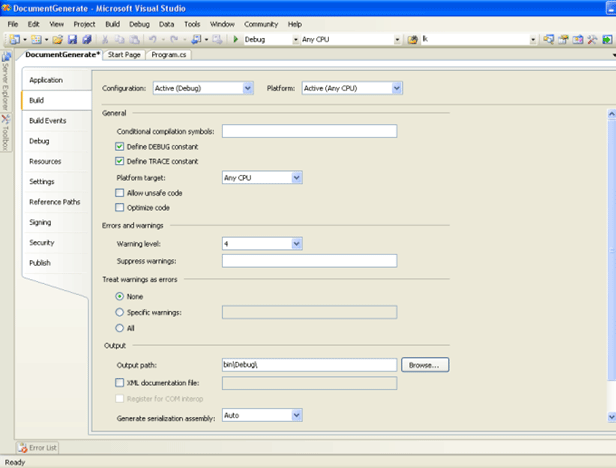Figure 3

3. Select the check box labeled XML documentation file as below: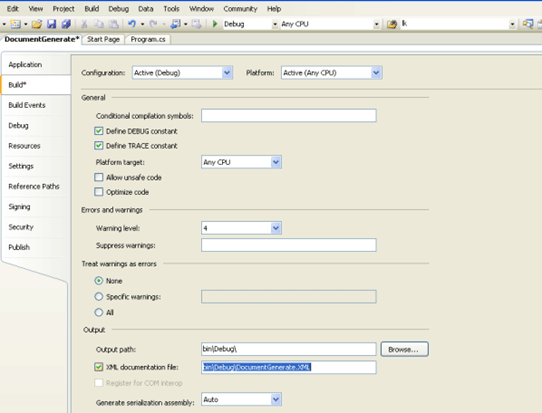Figure 4

You can precise the emplacement where the xml file will be generated.

4. Build the solution now pressing F6 then browse to the indicated xml file path, for my case I'll generate it in the bin\debug repertory as DocuementGenerate.xml

I give you a second way to achieve the same task but using the .Net command prompt this once:

1. Start>All programs then select the SDK command prompt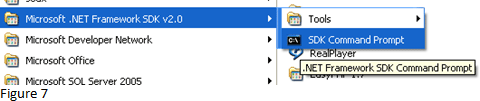Figure 5

In fact, there is an option within csc.exe tool the /doc. It enables to generate documentation for the project: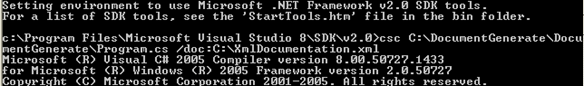Figure 6

I generate the documentation file in the C:\ directory this once using csc.exe tool, now, if you want to display the documentation contents to the console then type the command "type" followed by the xml documentation file full name as below: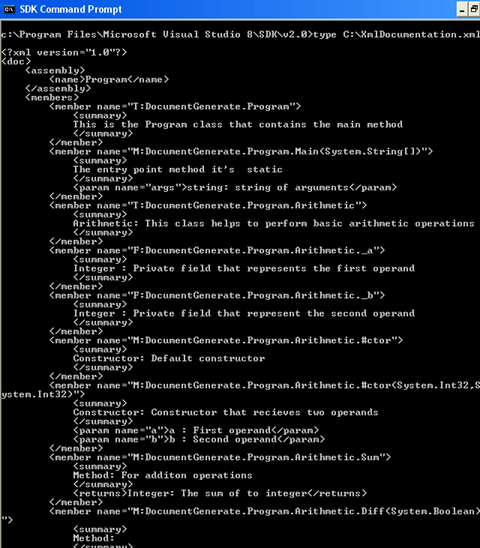Figure 7

As final step, we will generate an HTML document from the project documentation. To do so, we'll use XSL transformation to generate the output from the xml document. Let's say, we want to generate a particular documentation, we will list the summary contents as an ordered list.

Now, add a static method to the arithmetic class

static public void GenerateHTML(string XmlInputPath, string XslPath, string HTMLPath)

{

try

{

XslCompiledTransform oTransform = new XslCompiledTransform();

oTransform.Transform(XmlInputPath, HTMLPath);

Console.WriteLine("Html file generated");

}

catch (XsltCompileException caught)

{

Console.WriteLine("Xslt compile exception :" + caught.Message );

}

}

The arguments are the input xml document path, the xsl file path and the output html document.

XML Input:

The xml input in this case is C:\XmlDocumentation.xml

<?xml version="1.0"?>

<doc>

<assembly>

<name>Program</name>

</assembly>

<members>

<member name="T:DocumentGenerate.Program">

<summary>

This is the Program class that contains the main method

</summary>

</member>

<member name="M:DocumentGenerate.Program.Main(System.String[])">

<summary>

The entry point method it's  static

</summary>

<param name="args">string: string of arguments</param>

</member>

<member name="T:DocumentGenerate.Program.Arithmetic">

<summary>

Arithmetic: This class helps to perform basic arithmetic operations

</summary>

</member>

<member name="F:DocumentGenerate.Program.Arithmetic._a">

<summary>

Integer : Private field that represents the first operand

</summary>

</member>

<member name="F:DocumentGenerate.Program.Arithmetic._b">

<summary>

Integer : Private field that represent the second operand

</summary>

</member>

<member name="M:DocumentGenerate.Program.Arithmetic.#ctor">

<summary>

Constructor: Default constructor

</summary>

</member>

<member name="M:DocumentGenerate.Program.Arithmetic.#ctor(System.Int32,System.Int32)">

<summary>

Constructor: Constructor that recieves two operands

</summary>

<param name="a">a : First operand</param>

<param name="b">b : Second operand</param>

</member>

<member name="M:DocumentGenerate.Program.Arithmetic.Sum">

<summary>

</summary>

<returns>Integer: The sum of to integer</returns>

</member>

<member name="M:DocumentGenerate.Program.Arithmetic.Diff(System.Boolean)">

<summary>

Method:

</summary>

<param name="FirstMinusSecond">

Boolean : Indicates if

the a should be at first position

</param>

<returns>Integer: Difference between to integers</returns>

</member>

<member name="M:DocumentGenerate.Program.Arithmetic.Mult">

<summary>

Method : Multiply two integers

</summary>

<returns>Integer: Multiplication of two integers</returns>

</member>

<member name="M:DocumentGenerate.Program.Arithmetic.Div(System.Boolean)">

<summary>

Method : Divide one integer by another integer

</summary>

<param name="FirstDivSecond">

Boolean: Indicates if

the first operand should be a

</param>

<returns>Double: Division of two integers</returns>

</member>

<member name="P:DocumentGenerate.Program.Arithmetic.a">

<summary>

Integer: This is the first operand public propertie

</summary>

</member>

<member name="P:DocumentGenerate.Program.Arithmetic.b">

<summary>

Integer: This is the second operand public propertie

</summary>

</member>

</members>
</doc>

It is generated automatically using NDOC under Visual studio

XSL Transform:

This is the extensible transform file in this case is located in C:\TransformXmlDoc.xsl:

<?xml version="1.0" encoding="UTF-8" ?>

<xsl:stylesheet version="1.0" xmlns:xsl="http://www.w3.org/1999/XSL/Transform">

<xsl:template match="/">

<ol>

<xsl:for-each select="doc/members/member">

<li>

<xsl:value-of select="summary"/>

</li>

</xsl:for-each>

</ol>

</xsl:template>
</xsl:stylesheet>

Now call the GenerateHTML method from the main method.

//Generate the documentation

Arithmetic.GenerateHTML(@"C:\XmlDocumentation.xml",@"C:\TransformXmlDoc.xsl", @"C:\HtmlDocumentation.html");

Good dotneting !!!

Recommended Free Ebook
Similar Articles